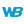• Drawing in Browser code
WINDEVWEBDEVWINDEV MobileOthersDraws a rectangle in an Image control.Caution: A specific configuration is required to use this function in Linux. For more details, see The drawings.
Example
// Draw a rectangle whose background changes from red to blue
// via yellow
IMG_Image1.DrawRectangleGradient(10, 20, 100, 150, LightRed, LightBlue, 0, LightYellow, 30)
Syntax

Drawing a rectangle by specifying the points of the rectangle and the gradient

<Image control>.DrawRectangleGradient(<X1> , <Y1> , <X2> , <Y2> , <Start color> , <End color> [, <Angle> [, <Color 3> [, <Distance color 3> [, <Color 4> [, <Distance color 4>]]]]])
<Image control>: Control name
Name of the Image control to be used.
<X1>: Integer
X-coordinate (in pixels) of the upper-left corner of the rectangle.
<Y1>: Integer
Y-coordinate (in pixels) of the upper-left corner of the rectangle.
<X2>: Integer
X-coordinate (in pixels) of the lower-right corner of the rectangle.
<Y2>: Integer
Y-coordinate (in pixels) of the lower-right corner of the rectangle.
<Start color>: Integer or constant
Start color of the gradient. This color can correspond to:
<End color>: Integer or constant
End color of the gradient. This color can correspond to:
<Angle>: Optional integer
Angle that will be used for the selection of colors in the gradient. The angle value is included between 0 (horizontal angle, default value) and 360°.Only the following angles can be used: 0,90,180,270,360.
<Color 3>: Optional constant or integer
Color that will be used in the gradient. This color can correspond to:This parameter is not available.
<Distance color 3>: Optional integer
Percentage separating the start color from color 3.This parameter is not available.
<Color 4>: Optional constant or integer
Color that will be used in the gradient. This color can correspond to:This parameter is not available.
<Distance color 4>: Optional integer
Percentage separating the start color from color 4.This parameter is not available.Drawing a rectangle by specifying the points of the rectangle and by using the Background type

<Image control>: Control name
Name of the Image control to be used.
<X1>: Integer
X-coordinate (in pixels) of the upper-left corner of the rectangle.
<Y1>: Integer
Y-coordinate (in pixels) of the upper-left corner of the rectangle.
<X2>: Integer
X-coordinate (in pixels) of the lower-right corner of the rectangle.
<Y2>: Integer
Y-coordinate (in pixels) of the lower-right corner of the rectangle.
Name of the Background variable the with the gradient characteristics.Drawing a rectangle by using the Rectangle type and by specifying the gradient

<Image control>.DrawRectangleGradient(<Rectangle> , <Start color> , <End color> [, <Angle> [, <Color 3> [, <Distance color 3> [, <Color 4> [, <Distance color 4>]]]]])
<Image control>: Control name
Name of the Image control to be used.
<Rectangle>: Rectangle variable
Name of the Rectangle variable that defines the coordinates of the rectangle to draw.
<Start color>: Integer or constant
Start color of the gradient. This color can correspond to:
<End color>: Integer or constant
End color of the gradient. This color can correspond to:
<Angle>: Optional integer
Angle that will be used for the selection of colors in the gradient. The angle value is included between 0 (horizontal angle, default value) and 360°.
<Color 3>: Optional constant or integer
Color that will be used in the gradient. This color can correspond to:
<Distance color 3>: Optional integer
Percentage separating the start color from color 3.
<Color 4>: Optional constant or integer
Color that will be used in the gradient. This color can correspond to:
<Distance color 4>: Optional integer
Percentage separating the start color from color 4.Drawing a rectangle by using the Rectangle type and the Background type

<Image control>: Control name
Name of the Image control to be used.
<Rectangle>: Rectangle variable
Name of the Rectangle variable that defines the coordinates of the rectangle to draw.
Name of the Background variable the with the gradient characteristics.
Remarks

The color parameters are used as follows:The angle is used to define the orientation of the gradient.Gradients with 3 or 4 colors are only available for 0 or 90 degree angles.
Remark: The gradient colors are not available for some systems (Windows 98 or Me for example) or for TSE 256 colors. In this case, a rectangle whose color corresponds to <Start color> is displayed.
Related Examples:Unit examples (WINDEV): The drawing functions [ + ] Using the main drawing functions of WINDEV to:- Initialize an Image control for drawing- Draw simple shapes- Write a text into a drawing- Change the color in a drawing
Business / UI classification: Neutral code
Component: wd280pnt.dll
Minimum version required
• Version 24